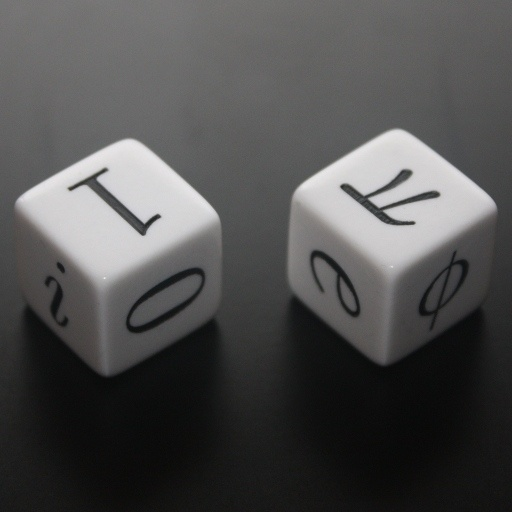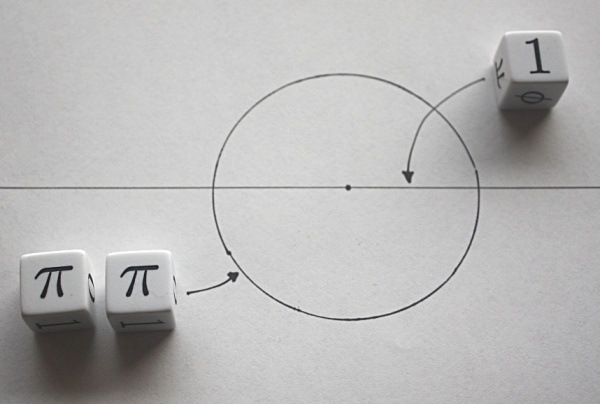# Mathematician’s DiceA lot of people I know hate math, but I don’t. It’s hard to hate if you studied five years to get an engineering degree which is obviously full of math courses. That doesn’t mean I’m over the moon over math; I just happen to like it, that’s all. And if you’re of the same opinion, then I’m sure you’ll find the Mathematician’s Dice pretty darn cool.

The Mathematician’s Dice have six of the most important numbers in math printed on each of its faces: the imaginary number i; the additive identity 0, the multiplicative identity 1, the golden ratio φ (1.618…); Euler’s number e (2.718…); and the circular constant π (3.141…).

Just like the Writer’s Block is for writers and Rory’s Story Cubes is for aspiring storytellers, the Mathematician’s Dice is for the aspiring mathematician.Mathematician’s Dice

The Mathematician’s Dice are dice designed for mathematicians! Rather than the relatively boring numbers 1, 2, 3, 4, 5, and 6, these dice are jam-packed with the six most important numbers in mathematics! They have: the imaginary number i; the additive identity 0, the multiplicative identity 1, the golden ratio φ (1.618…); Euler’s number e (2.718…); and the circular constant π (3.141…).

The Mathematician’s Dice is available online from Glyphobet for \$8.99 for one die, or \$17.98 for a pair of dice.